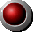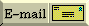# "Gallons of Ethanol"

 A one-gallon engine             A gallon of ethanol         ... takes a gallon of gasoline to produce General Motors produces a V-6 engine with 231 cubic-inch displacement. One gallon is defined to be 231 cubic inches, but that didn't cause GM to refer to their 6-banger as a one-gallon engine. One inch is defined to be 2.54 centimeters.  Therefore, one gallon is 231 X 2.54 X 2.54 X 2.54 = 3785.411784 cubic centimeters.  (All of those digits are legitimate, because it is a matter of definition, not measurement.)  GM's engine is thus a 3.8 liter (1 liter = 1000 cubic centimeters) engine. The "heat content" of a gallon of ethanol is 76,000 British Thermal Units (BTU).  But 1 BTU = 1054 joules, so the energy density (by volume) of ethanol is 21.2 kilojoules per cubic centimeter. Since the density of ethanol is 0.789 grams per cubic centimeter, the energy density of ethanol is 26.8 megajoules per kilogram.  This compares rather unfavorably with gasoline at 45 MJ per kilogram, but is rather appalingly low, when it takes the energy of a gallon of gasoline to produce the gallon of ethanol from corn!
To main Energy Advocate IndexThe Energy Advocate